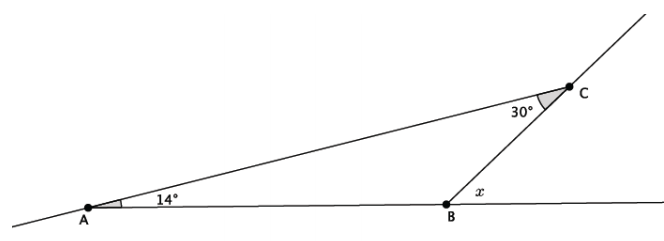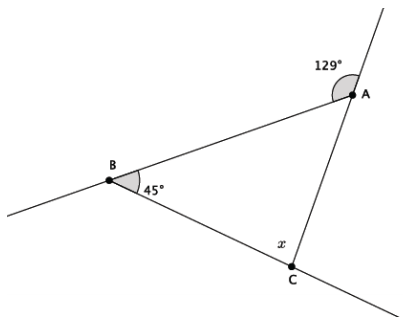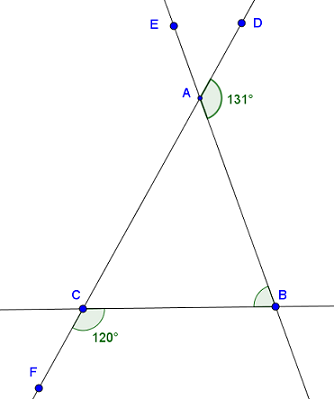# Transformations and Angle Relationships

## Objective

Define and use the exterior angle theorem for triangles.

## Common Core Standards

### Core Standards

?

• 8.G.A.5 — Use informal arguments to establish facts about the angle sum and exterior angle of triangles, about the angles created when parallel lines are cut by a transversal, and the angle-angle criterion for similarity of triangles. For example, arrange three copies of the same triangle so that the sum of the three angles appears to form a line, and give an argument in terms of transversals why this is so.

?

• 7.G.A.2

• 7.G.B.5

## Criteria for Success

?

1. Extend line segments of a polygon to see exterior angles.
2. Know that exterior angles are supplementary to the adjacent interior angle.
3. Know that exterior angles of a triangle are equal to the sum of the two opposite interior angles.
4. Use the exterior angle sum theorem and other properties of angles to determine additional angle relationships.

#### Fishtank Plus

• Problem Set
• Student Handout Editor
• Vocabulary Package

## Anchor Problems

?

### Problem 1

Triangle ${ABC}$ has two interior angles that measure ${14^{\circ}}$ and ${30^{\circ}}$.1. What is the measure of the exterior angle marked by $x$?
2. What is the relationship between the value of $x$ and the two given interior angles?

#### References

EngageNY Mathematics Grade 8 Mathematics > Module 2 > Topic C > Lesson 14Teacher Version: Example 1

Grade 8 Mathematics > Module 2 > Topic C > Lesson 14 of the New York State Common Core Mathematics Curriculum from EngageNY and Great Minds. © 2015 Great Minds. Licensed by EngageNY of the New York State Education Department under the CC BY-NC-SA 3.0 US license. Accessed Dec. 2, 2016, 5:15 p.m..

Modified by The Match Foundation, Inc.

### Problem 2

Triangle ${ABC}$ has an interior angle that measures ${45^{\circ}}$ and an exterior angle that measures ${129^{\circ}}$
What is the measure of the interior angle marked by $x$?#### References

EngageNY Mathematics Grade 8 Mathematics > Module 2 > Topic C > Lesson 14Teacher Version: Example 4

Grade 8 Mathematics > Module 2 > Topic C > Lesson 14 of the New York State Common Core Mathematics Curriculum from EngageNY and Great Minds. © 2015 Great Minds. Licensed by EngageNY of the New York State Education Department under the CC BY-NC-SA 3.0 US license. Accessed Dec. 2, 2016, 5:15 p.m..

Modified by The Match Foundation, Inc.

## Problem Set

?The following resources include problems and activities aligned to the objective of the lesson that can be used to create your own problem set.

Triangle ${ABC}$ is shown in the diagram below. Show two different ways that you can determine the measure of $\angle {ABC}$.